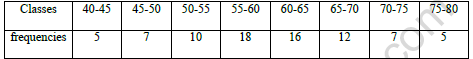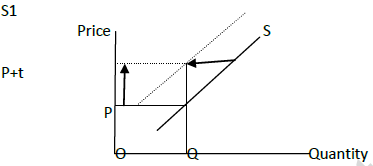# CBSE Class 11 Economics Worksheet Set E Solved

Download printable Economics Class 11 Worksheets in pdf format, CBSE Class 11 Economics Worksheet Set E Solved has been prepared as per the latest syllabus and exam pattern issued by CBSE, NCERT and KVS. Also download free pdf Economics Class 11 Assignments and practice them daily to get better marks in tests and exams for Grade 11. Free chapter wise worksheets with answers have been designed by Standard 11 teachers as per latest examination pattern

PART A

1. Price elasticity of supply is unitary. What could be the percentage change in quantity supplied if its price increases by 10 percent?

2. Define elasticity of supply

3. A downward movement along the same supply curve is due to:
(i) Increase in prices of inputs
(ii) Increase in unit taxes
(iii) Increase in price of substitute in production
(iv) Decrease in price of the good.

4. How does supply of a commodity change when government imposes a unit tax? Explain with diagram

5. Briefly explain the theory of firm’s equilibrium under conditions of perfect competition. Use diagram.

SECTION B

6. Define third quartile.

7. State one area in which mode is widely used.

8. Second quartile is also called:
(i) Mean
(ii) Range
(iii) Mode
(iv) Median

9. Find lower quartile and upper quartile from the following

Values: 9   15   6   12   20   18  8   11   13   10   14

10. Locate Value of mode on a graph and verify the resultVALUE POINTS

1. 10% Increase

2. Degree of responsiveness of quantity demanded for a given change in price of the good

3. (iv) Decrease in price of the good.

4. Init tax will increase MC. Less units only can be supplied at the same price. MC curve shift up. Supply curve will shift to left. Supply decreases.5. A profit maximizing firm will produce that many numbers of units at which profit is maximum. Profit is maximum when TR-TC is maximum. TR-TC is maximum when two conditions are achieved.

a. MR must be equal to MC at profit maximum output

b. MC must be non diminishing at profit maximum output

If MR>MC, increase in TR is more than increase in TC when firm increases output. Profit is increasing when firm increases output. Firm is not at equilibrium because it wants to increase output.

If MR<MC, increase in TR is less than increase in TC when firm increases output. Profit diminishes when firm increases output. In other words profit will increase when firm reduces output. Firm is not at equilibrium because it wants to reduce
output.

This means Firm makes maximum profits when MR=MC. Firm is at equilibriumAt point A MC=MR but MC is falling so that firm is not at equilibrium
At output Q1, MR>MC, so that firm will increase the output
At output level Q3 Mc>MR, firm will reduce output
At Output Q2 Firm is at equilibrium because MC=MR and MC is falling.

PART B

6. Quartiles are values that divide the arranged series in to four equal parts

7. Quality control

8. (iv) Median

9. Arrange values in ascending order.

6, 8, 9, 10, 11, 12, 13, 14, 15, 18, 20

Q1= (N+1)/4 the item; 11+1/4 = 12/4 = 3rd item. Q1= 9
Q2 = 2(N+1)/4 the item. 2(11+1)/4; 6th item. Q2 = 12
Q3 = 3((N+1)/4 the item 3(11+1)/4; 9th item. Q3 = 15

10. Mode = l + (fm-f1)/(2xfm-f1-f2) x h
=55 + (18-10)/(2x18-10-16) x5
=55 +4 = 59
Histogram and location

## Tags:

Click for more Economics Study Material
 CBSE Class 11 Economics Arithmetic Mean And Weighted Mean Worksheet CBSE Class 11 Economics Collection of Data Worksheet Set A CBSE Class 11 Economics Collection of Data Worksheet Set B CBSE Class 11 Economics Collection of Data Worksheet Set C CBSE Class 11 Economics Comparative Development Experience Worksheet CBSE Class 11 Economics Demand Analysis Worksheet Set A CBSE Class 11 Economics Elasticity of Demand Worksheet CBSE Class 11 Economics Indefference Curve Analysis Worksheet CBSE Class 11 Economics Index Number Worksheet CBSE Class 11 Economics Indian Economy On The Eve Of Independence Worksheet CBSE Class 11 Economics Indian Economy Worksheet CBSE Class 11 Economics Introduction to Microeconomics Worksheet CBSE Class 11 Economics Introduction Worksheet CBSE Class 11 Economics Introduction Worksheet Set A CBSE Class 11 Economics Market Equilibrium Worksheet CBSE Class 11 Economics Median And Quartiles Worksheet CBSE Class 11 Economics Non Competitive Market Worksheet CBSE Class 11 Economics On Employment Worksheet CBSE Class 11 Economics On Human Capital Formation Worksheet CBSE Class 11 Economics On Median And Quartiles Worksheet CBSE Class 11 Economics On Poverty Worksheet CBSE Class 11 Economics On Presentation of Data Worksheet CBSE Class 11 Economics On Range and Quartile Deviation Worksheet CBSE Class 11 Economics On Rural Development Worksheet CBSE Class 11 Economics Organisation of Data Worksheet Set A CBSE Class 11 Economics Organisation of Data Worksheet Set B CBSE Class 11 Economics Organisation of Data Worksheet Set C CBSE Class 11 Economics Organisation of Data Worksheet Set D CBSE Class 11 Economics Presentation of data Worksheet CBSE Class 11 Economics Production And Cost Worksheet CBSE Class 11 Economics Production Function Worksheet CBSE Class 11 Economics Production Possibility Curve Worksheet CBSE Class 11 Economics Statics For Economics Worksheet Set A CBSE Class 11 Economics Statics For Economics Worksheet Set B CBSE Class 11 Economics Statics For Economics Worksheet Set C CBSE Class 11 Economics Statics Introduction Worksheet CBSE Class 11 Economics Statistics For Economics Worksheet CBSE Class 11 Economics Theory of Firm Under Perfect Competition Worksheet CBSE Class 11 Economics Utility Analysis Worksheet CBSE Class 11 Economics Worksheet Set A Solved CBSE Class 11 Economics Worksheet Set B Solved CBSE Class 11 Economics Worksheet Set C Solved CBSE Class 11 Economics Worksheet Set D Solved CBSE Class 11 Economics Worksheet Set E Solved CBSE Class 11 Economics Worksheet Set F Solved CBSE Class 11 Economics Worksheet Set G Solved CBSE Class 11 Economics Worksheet Set H Solved CBSE Class 11 Economics Worksheet Set I Solved CBSE Class 11 Economics Worksheet Set J Solved CBSE Class 11 Economics Worksheet Set K Solved CBSE Class 11 Economics Worksheet Set L Solved CBSE Class 11 Micro Economics Consumer Behaviour And Demand Analysis Worksheet CBSE Class 11 Micro Economics Consumer Behaviour And Demand Indifference Analysis Worksheet Set A CBSE Class 11 Micro Economics Consumer Behaviour And Demand Utility Analysis Worksheet CBSE Class 11 Micro Economics Indifference Curve Analysis Worksheet CBSE Class 11 Micro Economics Introduction Worksheet CBSE Class 11 Micro Economics Price Elasticity of Demand Worksheet CBSE Class 11 Micro Economics Theory of Demand Worksheet

## Latest NCERT & CBSE News

Read the latest news and announcements from NCERT and CBSE below. Important updates relating to your studies which will help you to keep yourself updated with latest happenings in school level education. Keep yourself updated with all latest news and also read articles from teachers which will help you to improve your studies, increase motivation level and promote faster learning

### FAQs on Policy for Tabulation of Marks for Class 10 Board Exams

ON POLICY Question.1 How CBSE will declare the result of Class-X? Answer. Results of Class X Board will be declared on the basis of an objective criterion developed by the Board vide Notification no.CBSE/CE/2021 dated 01.05.2021. Question.2. If any candidate is not...

### Moderation of Marks Class 11 and 12 Board Exams

The portal for moderation and finalization of results for Class-12 is being opened from 16.07.2021 to 22.07.2021. As Board has to declare the result latest by 31.07.2021, schools have been requested to follow the schedule strictly and complete the moderation within...

### CBSE Class 12 Evaluation Criteria 2021

SUMMARY OF THE POLICY FOR TABULATION OF MARKS FOR CLASS XII BOARD EXAMINATIONS 2021   1(a) Due to cancellation of the Board examinations, the assessment of theory portion of 80/70/60/50/30 marks will be done by the school based on the following:     Class...

### CBSE Board Examination Scheme of Assessment 2021 2022

A. Academic session to be divided into 2 Terms with approximately 50% syllabus in each term: The syllabus for the Academic session 2021-22 will be divided into 2 terms by following a systematic approach by looking into the interconnectivity of concepts and topics by...